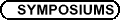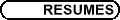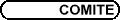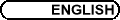CMS Doctoral Prize Lecture / Conférence du Prix de doctorat de la SMC NICOLAAS SPRONK, University of Waterloo, Waterloo, ON N2L 3G1 Operator spaces and abstract harmonic analysis If G is a locally compact abelian group, there is another locally compact abelian group, the Pontryagin dual group [^(G)], which characterises G in the sense that [^([^(G)])] is homeomorphically isomorphic with G. In studying the algebraic and topological properties of G, it is frequently necessary to study its group algebra L1(G), the algebra of Haar integrable functions on G. In the case that G has discrete topology this algebra contains the group ring C[G]; exactly as the algebra of Laurent series with summable coefficients contains the algebra of Laurent polynomials C[Z]. If G is not abelian, there is often no object which directly plays as rich a role as does [^(G)] from the abelain case. However, there is a construction of an abelian Banach algebra by Eymard, the Fourier algebra A(G), which in the abelian case is exactly L1([^(G)]). Categorically, A(G) plays the role of "[^(G)]". Although A(G) is ostensibly commutative, spatially it is not commutative. The theory of operator spaces is thus required to understand the actual structure of A(G), in its capacity as a dual object of G. I will explain some background on this subject, and highlight results of mine and of many leading researchers, which show that the operator space structure is indispensible in our modern understanding of A(G). In particular I want to note recent achievements in understanding cohomological properties, ideals, multipliers and homomomorphisms.